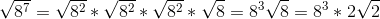Example Questions

Example Question #53 : Basic Squaring / Square Roots

Simplify the following: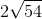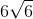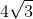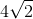Explanation:

To solve, you must first break up 54 into its smallest prime factors. Those are: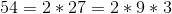Since our root has index 2, that means that for every 2 identical factors inside, you can pull 1 out. Thus, we get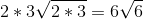Example Question #54 : Basic Squaring / Square Roots

Simplify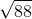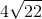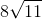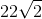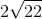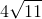Explanation:

To simplify a square root, we need to find perfect squares. In this case, it is.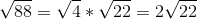Example Question #55 : Basic Squaring / Square Roots

Simplify: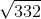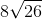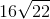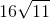Explanation:

To simplify a square root, we need to find perfect squares. In this case, it is.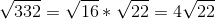Example Question #56 : Basic Squaring / Square Roots

Simplify: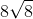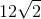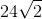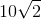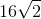Explanation:

To simplify a square root, we need to find perfect squares. In this case, it is. Since there is a number outside the radical, we ignore that for now and later we multiply the number and square root.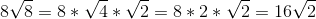Example Question #57 : Basic Squaring / Square Roots

Simplify: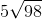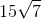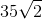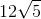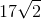Explanation:

To simplify a square root, we need to find perfect squares. In this case, it is. Since there is a number outside the radical, we ignore that for now and later we multiply the number and square root.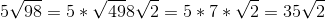Example Question #58 : Basic Squaring / Square Roots

Simplify: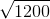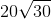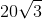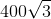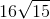Explanation:

To simplify radicals, we need to find a perfect square to factor out. In this case, its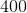.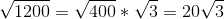Example Question #59 : Basic Squaring / Square Roots

Simplify: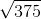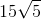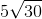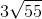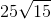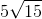Explanation:

To simplify radicals, we need to find a perfect square to factor out. In this case, its.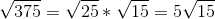Example Question #60 : Basic Squaring / Square Roots

Simplify: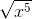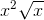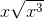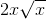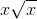Explanation:

To solve this, we know perfect squares are able to simplify easily to the base it is. Let's find all the perfect squares in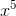.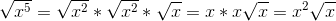Example Question #61 : Basic Squaring / Square Roots

Simplify: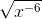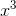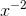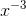It's impossible because the value is negative.Explanation:

Although the exponent is negative, we know that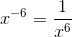. Therefore, we have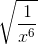. Let's simplify this by finding perfect squares.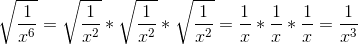Example Question #62 : Basic Squaring / Square Roots

Simplify: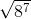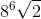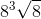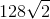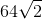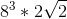To solve this, we know perfect squares are able to simplify easily to the base it is. Let's find all the perfect squares in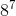.